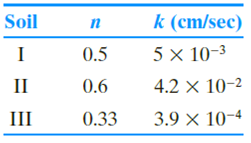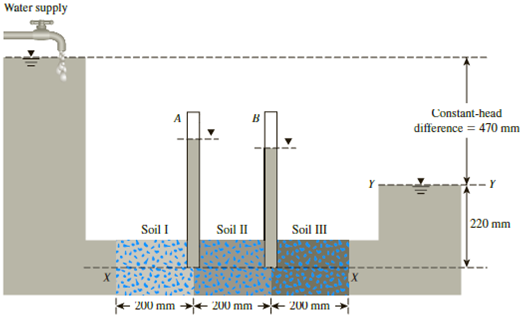Chapter 7, Problem 7.1CTPPrinciples of Geotechnical Enginee...

9th Edition
Braja M. Das + 1 other
ISBN: 9781305970939

Solutions

Chapter
SectionPrinciples of Geotechnical Enginee...

9th Edition
Braja M. Das + 1 other
ISBN: 9781305970939
Textbook Problem

Section 7.2 described the importance of total head and hydraulic gradient on the seepage of water through permeable soil media. In this problem, we will study the variations of head along the axis of a soil specimen through which seepage is occurring. Consider the setup shown in Figure 7.34 (similar to Example 7.15) in which three different soil layers, each 200 mm in length, are located inside a cylindrical tube of diameter 150 mm. A constant-head difference of 470 mm is maintained across the soil sample. The porosities and hydraulic conductivities of the three soils in the direction of the flow are given here.Perform the following tasks.a. Determine the quantity of water flowing through the sample per hour.b. Denoting the downstream water level (Y–Y) to be the datum, determine the elevation head (Z), pressure head (u/γw) and the total head (h) at the entrance and exit of each soil layer.c. Plot the variation of the elevation head, pressure head and the total head with the horizontal distance along the sample axis (X–X).d. Plot the variations of discharge velocity and the seepage velocity along the sample axis.e. What will be the height of the vertical columns of water inside piezometers A and B installed on the sample axis?Figure 7.34

(a)

To determine

Find the quantity of water flowing through the sample per hour.

Explanation

Given information:

The length of each soil layer (H1,H2,H3) is 200 mm.

The total length of the soil layer H is 600 mm.

The diameter of the cylindrical tube d is 150 mm.

The constant head difference Δh is 470 mm.

The porosity of the soil layer I (n1) is 0.5.

The porosity of the soil layer II (n2) is 0.6.

The porosity of the soil layer III (n3) is 0.33.

The hydraulic conductivity of soil layer I (k1) is 5×103cm/sec.

The hydraulic conductivity of soil layer II (k2) is 4.2×102cm/sec.

The hydraulic conductivity of soil layer III (k3) is 3.9×104cm/sec.

Calculation:

Determine the hydraulic conductivity in the vertical direction using the relation.

kV(eq)=HH1k1+H2k2+H3k3

Substitute 600 mm for H, 200 mm for H1, 5×103cm/sec for k1, 200 mm for H2, 4.2×102cm/sec for k2, 200 mm for H3, and 3.9×104cm/sec for k3.

kV(eq)=600mm×1cm10mm200mm×1cm10mm5×103+200mm×1cm10mm4

(b)

To determine

Find the elevation head (Z), pressure head (u/γw), and the total head (h) at the entrance and exit of the each soil layer.

(c)

To determine

Plot the variation of the elevation head, pressure head, and the total head with the horizontal distance along the sample axis (X–X).

(d)

To determine

Plot the variation of the discharge velocity and the seepage velocity along the sample axis (X–X).

(e)

To determine

Find the height of the vertical columns of water inside piezometers A and B installed on the sample axis.

Still sussing out bartleby?

Check out a sample textbook solution.

See a sample solution

The Solution to Your Study Problems

Bartleby provides explanations to thousands of textbook problems written by our experts, many with advanced degrees!

Get Started

Describe parasitic draw.

Automotive Technology: A Systems Approach (MindTap Course List)

How are network footprinting and network fingerprinting related?

Principles of Information Security (MindTap Course List)

What is an advantage of using locking pliers?

Precision Machining Technology (MindTap Course List)

Why is an object said to have greater semantic content than an entity?

Database Systems: Design, Implementation, & Management

What are the main components of a database system?

Database Systems: Design, Implementation, & Management

What strategies e Wal-Mart and Loweâ€™s using to gain more online customers?

Systems Analysis and Design (Shelly Cashman Series) (MindTap Course List)

Use A992 steal and. select a W shape. a. Use LRFD. b. Use ASD.

Steel Design (Activate Learning with these NEW titles from Engineering!)